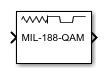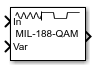# MIL-188 QAM Demodulator Baseband

MIL-STD-188-110 B/C standard-specific quadrature amplitude demodulation

Since R2018b

•Libraries:
Communications Toolbox / Modulation / Digital Baseband Modulation / AM
Communications Toolbox / Modulation / Digital Baseband Modulation / Standard-Compliant

## Description

The MIL-188 QAM Demodulator Baseband block demodulates the input signal using MIL-STD-188-110 standard-specific quadrature amplitude modulation (QAM). For a description of MIL-STD-188 compliant demodulation, see MIL-STD-188-110 QAM Hard Demodulation and MIL-STD-188-110 QAM Soft Demodulation.

This icon shows the block with all ports enabled:## Ports

### Input

expand all

MIL-STD-188 standard-specific QAM modulated signal, specified as a scalar, vector, or matrix. When this input is a matrix, each column is treated as an independent channel. This port is unnamed until the `Var` port is enabled.

Data Types: `single` | `double`
Complex Number Support: Yes

Noise variance, specified as a positive scalar or vector of positive values. When the noise variance or signal power result in computations involving extreme positive or negative magnitudes, see MIL-STD-188-110 QAM Soft Demodulation for demodulation decision type considerations.

#### Dependencies

To enable this port set the Noise variance source parameter to `Input port`.

### Output

expand all

Demodulated signal, returned as a scalar, vector, or matrix. The dimensions of the demodulated signal depend on the specified Output type and Decision type parameter values. This port is unnamed on the block.

Output typeDecision typeDemodulated Signal DescriptionDimensions of Demodulated Signal
`Integer`Demodulated integer values in the range [0, (M – 1)]The output signal has the same dimensions as input signal.
`Bit``Hard decision`Demodulated bitsThe number of rows in the output signal is log2(M) times the number of rows in the input signal. Each demodulated symbol is mapped to a group of log2(M) elements in a column, where the first element represents the MSB, and the last element represents the LSB.
```Log-likelihood ratio```Log-likelihood ratio value for each bit
```Approximate log-likelihood ratio```Approximate log-likelihood ratio value for each bit

M is the value of Modulation order.

Use Output data type to specify the output data type.

## Parameters

expand all

Modulation order, M, specified as `16`, `32`, `64`, or `256`. The modulation order specifies the total number of points in the constellation of the input signal.

Constellation scaling preference, specified as:

• `As specified in standard` – The block scales the constellation based on specifications in the relevant standard .

• `Unit average power` – The block scales the constellation to an average power of 1 watt referenced to 1 ohm.

Output type, specified as `Integer` or `Bit`. To use `Integer`, the input signal must consist of integers in the range [0, (M – 1)]. To use `Bit`, the input signal must contain binary values, and the number of rows must be an integer multiple of log2(M), where M is the Modulation order.

Demodulation decision type, specified as ```Hard decision```, `Log-likelihood ratio`, or `Approximate log-likelihood ratio`. See MIL-STD-188-110 QAM Soft Demodulation for algorithm selection considerations.

#### Dependencies

This parameter applies when Output type is set to `Bit`.

Noise variance source, specified as:

• `Property` — The noise variance is set using the Noise variance parameter.

• `Input port` — The noise variance is set using the Var input port.

#### Dependencies

This parameter applies only when Decision type is set to either `Log-likelihood ratio` or `Approximate log-likelihood ratio`.

Noise variance, specified as a positive scalar or vector of positive values.

• When specified as a scalar, that value is used on all elements in the input signal.

• When specified as a vector, the vector length must be equal to the number of columns in the input signal. Each noise variance vector element is applied to its corresponding column in the input signal.

When the noise variance or signal power result in computations involving extreme positive or negative magnitudes, see MIL-STD-188-110 QAM Soft Demodulation for demodulation decision type considerations.

#### Dependencies

This parameter applies only when Noise variance is set to `Property` and Decision type is set to either `Log-likelihood ratio` or `Approximate log-likelihood ratio`.

Output data type, specified as one of the acceptable values from this table. Acceptable Output data type values depend on the Output type and Decision type parameter values.

Output typeDecision typeOutput data type Options
`Integer`Not applicable`double`, `single`, `int8`, `uint8`, `int16`, `uint16`, `int32`, or `uint32`
`Bit``Hard decision``double`, `single`, `int8`, `uint8`, `int16`, `uint16`, `int32`, `uint32`, or `logical`
`Log-likelihood ratio` or ```Approximate log-likelihood ratio```The output signal is the same data type as the input signal.

#### Dependencies

This parameter applies only when Output type is set to `Integer` or when Output type is set to `Bit` and Decision type is set to ```Hard decision```.

Type of simulation to run, specified as ```Interpreted execution``` or `Code generation`.

• `Interpreted execution` — Simulate the model by using the MATLAB® interpreter. This option requires less startup time, but the speed of subsequent simulations is slower than with the ```Code generation``` option. In this mode, you can debug the source code of the block.

• `Code generation` — Simulate the model by using generated C code. The first time you run a simulation, Simulink® generates C code for the block. The model reuses the C code for subsequent simulations unless the model changes. This option requires additional startup time, but the speed of the subsequent simulations is faster than with the `Interpreted execution` option.

## Block Characteristics

 Data Types `Boolean` | `double` | `integer` | `single` Multidimensional Signals `yes` Variable-Size Signals `no`

• `Code generation` when using hard decision demodulation.
• `Interpreted execution` when using soft decision demodulation.